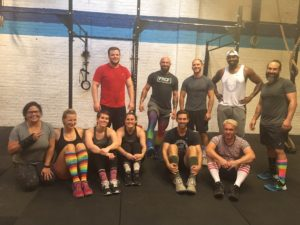## Friday, August 18, 2017

### Friday, August 18, 2017as many rounds as possible in 10 minutes:
50x squat
15x handstand pushup

### 1 Comment

1.–Scores–
Donal O.: 2 rounds + 3x hspu (sub: 1/3x hspu + 1/3x hspu abmat)
Anna G.: 4 rounds + 42x squat (sub: 2/3x strict bodyrow)
Alex M.: 5 rounds + 3x squat (sub: 2/3x ring dip)
Charles B.: 3 rounds + 1x wall climb
Hari P.: 4 rounds + 2x squat (sub: 1/2x hpsu)
Brian M.: 3 rounds + 10x squat (sub: 1/2x hspu abmat)
Michael F.: 4 rounds + 6x squat
Kat S.: 3 rounds + 31x squat (sub: 7/15x prom hspu)
Chris C.: 3 rounds + 5x hspu (sub: 200m row, strict)
Christopher J.: 3 rounds + 33x squat (sub; 2/3x atomic)
Sean F.: 3 rounds + 50x squat (sub: 2/3x hspu)
David G.: 3 rounds + 18x squat (sub: 7/15x hspu)
Thomas R.: 3 rounds + 1x hspu (sub: 7/15x hspu)
Aaron B.: 3 rounds + 9x atomic (sub: 2/3x kneeling atomic)
Diana G.: 3 rounds + 33x squat (sub: 2/3x atomic)
Carly B.: 3 rounds + 28x squat (sub: 2/3x kneeling atomic)
Charlie K.: 2 rounds + 35x squat (sub: 2/3x prom hspu)
Jerry I.: 3 rounds + 6x squat (sub: 2/3x atomic)
Drew P.: 4 rounds + 28x squat
JP S.: 3 rounds + 50x squat (sub: 7/15x prom hspu)
RJ A.: 3 rounds + 8x squat (sub: 2/3x atomic)
Matt S.: 3 rounds + 20x squat (sub: 7/15x hspu)
Alex Z.: 3 rounds + 4x squat (sub: 1/3x hspu)
Elise C.: 4 rounds + 29x squat (sub: 7/15x pushup)
Dan A.: 3 rounds + 23x squat (sub: 2/3x atomic)
Greg K.: 3 rounds + 36x squat (sub: 1/3x hspu)
Jen M.: 4 rounds + 10x squat (sub: 3/5x squat) 1/3x pushup)
Dave R.: 4 rounds + 28x squat
Chris H.: 4 rounds + 14x squat
Katherine C.: 3 rounds + 10x squat (sub: 3/5x squat to med ball, 2/3x kneeling atomic)
Bill M.: 3 rounds + 32x squat (sub: 7/15x hspu/hspu abmat)
Lisa C.: 4 rounds + 14x squat (sub: 2/3x strict bodyrow)
Edgar O.: 3 rounds (35-lb. dbs; sub: seated shoulder press)
Cassandra S.: 3 rounds + 44x squat (sub: 7/15x prom hspu)
Mark Ab.: 4 rounds (sub: 2/3x atomic)
Sean Mc.: 3 rounds + 35x squat (sub: 2/3x hspu)
Ralph B.: 4 rounds + 30x squat (sub: 1/3x hspu)
Travers O.: 4 rounds (sub: 1/3x prom hspu)
Adrienne V.: 3 rounds + 32x squat (sub: kneeling atomic)
Josh M.: 4 rounds + 23x squat
Jesse M.: 3 rounds + 34x squat (sub: 7/15x kneeling atomic)
Rachel B.: 4 rounds + 7x squat (sub: 7/15x kneeling atomic)
Mark Co.: 5 rounds + 3x squat (sub: 1/3x hspu)
Tara S.: 4 rounds + 46x squat (sub: push up)
Ross B.: 4 rounds + 21x squat (sub: 7/15x atomic)
Liz L.: 4 rounds + 3x squat (sub: kneeling atomic)
James H.: 3 rounds + 182m row (sub: 200m row)
Julian G.: 2 rounds + 30x squat (sub: 7/15x atomic)